﻿ Modal Analysis Using the Signal Processing Toolbox of Matlab 2017Publications are Open
Access in this journal
Article Versions
Export Article
• Normal Style
• MLA Style
• APA Style
• Chicago Style
Research Article
Open Access Peer-reviewed

### Modal Analysis Using the Signal Processing Toolbox of Matlab 2017

Peter Pavelka , Róbert Huňady, Martin Kučinský
American Journal of Mechanical Engineering. 2017, 5(6), 312-315. DOI: 10.12691/ajme-5-6-16
Published online: December 15, 2017

### Abstract

The paper deals with determination of modal parameters by two software applications. Experimental modal analysis was performed by the commercial system Pulse® designed for vibration analysis of mechanical structures. The system was used to obtain excitation signals and corresponding response signals. The measurement data were subsequently processed in Pulse ReflexTM software and Matlab 2017, of which the signal processing toolbox provides the new functionalities specially aimed for modal analysis. The toolbox contains functions to calculate frequency response functions and to estimate modal parameters. The aim of the article is to compare the results achieved by both softwares when the first approach realized using commercial system is considered as reference. The subject of this work is to evaluate the validity of the obtained results and to point out the possibilities that the new Matlab toolbox provides.

### 1. Introduction

Modal analysis has a wide application in solving the problems of engineering practice. Its application is mainly found in the automotive and aerospace industries or in the manufacture of domestic appliances.

Modal analysis is a process of determining modal parameters of mechanical systems. Modal parameters describe their dynamic behavior. In general, modal analysis can be theoretical or numerical and experimental. Theoretical approach deals with mathematical model of mechanical system and solves the eigenvalue problem. When the modal parameters are determined by measurement it is called experimental approach 1, 2, 3.

The basis of experimental modal analysis is to record the time histories of excitation force and corresponding response of an investigated object. Its dynamic behavior can be described in frequency domain by the frequency response function FRF or in time domain by impulse response function IRF. These functions are calculated from measurement data. The relation between FRF and IRF is defined by inverse Fourier Transform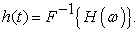(1)

Frequency response function is defined as the ratio of output signal to input signal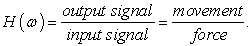(2)

There are three basic forms of FRFs depending on the type of response parameter, which can be either displacement or velocity or acceleration, see Table 1. Set of all FRFs is called frequency response function matrix. Definition of one element of FRF matrix for receptance is as follows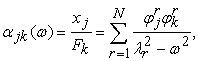(3)

where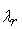is eigenvalue of the r-th mode (natural frequency + modal damping),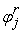is he j-th element of the r-th natural shapes' vector {Φ}, i.e. relative displacement at the j-th point as vibrating with the r-th shape, N is number of modes. 2

The vibration modes are determined from FRF or IRF by applying special estimation procedures. Each mode is characterized by natural frequency, damping ratio and mode shape. These characteristics are commonly called moda parameters.

### 2. Experimental Modal Analysis with Pulse®

Modal analysis was performed by Pulse® system. The investigated object was a steel rod with diameter 22 mm and length 800 mm (Figure 1). The responses were measured by piezoelectric uniaxial accerelerometer Brüel & Kjær type B4507 and object was excited by Brüel & Kjær impact hammer type 8206.

• Figure 1. Investigated object

The geometric model consisting of 18 excitation points (marked by black-green hammer) and one response point (marked by red arrow) is shown in Figure 2. These points are referred as degrees of freedom (DOF). The SIMO measurement was performed. The rod was freely supported using soft foam. Frequency range was set up to 10 kHz with length of 6400 samples and sampling frequency 25.6 kHz. Only bening modes in the direction of measurement were considered.

• Figure 2 Geometry with DOFs

The measurement was evaluated in commercial Pulse ReflexTM software. The obtained FRFs in the form of inertance are shown in Figure 3.

• Figure 3. FRFs obtained by Pulse ReflexTM

The Rational Fraction Polynomial method was used to estimate modal parameters. The natural frequencies and damping ratios for each identified mode are listed in Table 2. Figure 4 – Figure 15 show the corresponding mode shapes. It is clear that the mode shapes are more complicated as the natural frequency increases. Therefore, more DOFs are needed for a better shape representation of higher modes.

• Figure 4. First mode shape
• Figure 5. Second mode shape
• Figure 6. Third mode shape
• Figure 7. Fourth mode shape
• Figure 8. Fifth mode shape
• Figure 9. Sixth mode shape
• Figure 10. Seventh mode shape
• Figure 11. Eighth mode shape
• Figure 13. Tenth mode shape
• Figure 14. Eleventh mode shape
• Figure 15. Twelfth mode shape

The above results were considered to be references. The excitation time signals and response time signals were subsequently exported to wav format. The exported data was then imported to Matlab 2017.

### 3. Modal Analysis Using Matlab 2017

Singal Proccesissing Toolbox availabled in Matlab 2017 includes modal analysis functions that have been used to process the data exported from Pulse.

The function modalfrf was used to compile FRF matrix. Its syntax is as follows: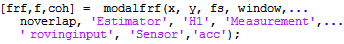The function modalfrf calulates a FRF matrix from the excitation signals (x) and the response signals (y), all sampled at a rate fs. The data of signal x and signal y must have the same number of rows. If x or y is a matrix, each column represents a signal. The signals can be multiplied by weighting window function (window). Matlab has a wide offer of window functions.

The output, frf, is an H1 estimate computed using Welch's method. Available are other method to estimate FRF, H2 and Hv. H1 method is used when there is noise at the input signal. H2 method is used when there is noise at the output signal. Hv method is used when there is noise at the input and output signal. The estimate method is determined by a comma-separated pair‚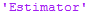,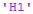or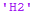or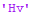. The defaut is H1 method. The comma-separated pair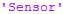,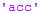or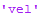or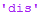is used to specifie the response parameter 1, 4.

The function modalfit is used for the estimation of modal parameters from FRFs. Its syntax is as follows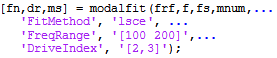The function modalfit estimates the natural frequencies, damping ratios and mode shapes vectors of modes (mnum = number of modes expected in the frequency range) of a system having measured frequency response functions (frf) defined at frequencies (f) and for a sample rate (fs). Least-Square Complex Exponential Methods and Peak Picking Methods are available to estimate modal parameters. The estimation method is determined by a comma-separated pair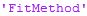,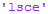or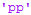. The estimation in a specific frequency range, e.g. from 100 to 200 Hz, is adjust by a comma-separated pair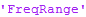,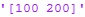. 4

The modalfrf function was used to create a frequency response function for all measured combination excitation/response. The obtained FRFs are shown in Figure 16.

• Figure 16. FRFs obtained from measured signals using modalfrf function

As can be seen, the functions are different from the references. Therefore, the function tfestimate that uses Welch's averaged periodogram method was used to compute new FRFs from measured data. Its syntax is as follows: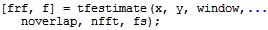where (nfft) defines the number of sampling points to calculate the discrete Fourier transform. The FRFs obtained such way are shown in Figure 17. An increased noise level may be observed, but sufficient match is achieved in the resonance areas.

• Figure 17. FRFs obtained from measured signals by the standard procedure using Fast Fourier Transform (FFT) algorithm

The estimation of modal parameters in Matlab was carried out by the function modalfit which was applied to four frequency ranges 0 ÷ 1 kHz, 1 ÷ 3 kHz, 3 ÷ 5 kHz, 5 ÷ 7.5 kHz, 7.5 ÷ 10 kHz, respectively. In this case, the PP method provides better estimation than the LSCE method, especially in terms of damping values. The estimated modal parameters are shown in Table 3.

The comparison of the results listed in Table 2 and Table 3 shows the sufficient match in natural frequencies and damping ratios. Mode shapes identified by modalfit function are shown in Figure 18 and Figure 19.

• Figure 18. Mode shapes (1-6) estimated by Matlab functions
• Figure 19. Mode shapes (7-12) estimated by Matlab functions

### 4. Conclusion

In the paper, the experimental modal analysis of the free supported steel rod was performed for the purpose of the assessment the accuracy of the results obtained by using the new Matlab functions aimed to estimation of modal parameters. The function modalfrf was used to calculate frequency response functions, i.e. to built FRF matrix from time signals of excitation and response. FRFs obtained using these function were not correct compared to the references. Therefore, the older function tfestimate had to be used instead. It is necessary to note that the change of parameter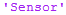to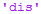solved the problem, but this setting did not correspond to reality. The function modalfit was used for the estimation of modal parameters from FRF matrix. The function uses two methods (PP, LSCE) for the estimation. Since the both methods have its advantages and disadvantages, the emphasis must be placed on their use and interpretation of the results.

### Acknowledgements

This work was supported by project VEGA 1/0393/14.

### References

  Zhi-Sai Ma, Li Liu, Si-Da Zhou, Di Jiang, Yuan-Yuan He, “Effects of Bolted Connection on Beam Structural Modal Parameters” in Topics in Dynamics of Bridges, Volume 3, Kluwer Academic Publishers. In article View Article  Bilošova, A., Modal Testing, VŠB TU Ostrava, 2011. In article  Ewins D. J. Modal testing – Theory, practice and application. 2. edition, Wiley, (2000). In article  Matlab 2017 help. In articleThis work is licensed under a Creative Commons Attribution 4.0 International License. To view a copy of this license, visit http://creativecommons.org/licenses/by/4.0/